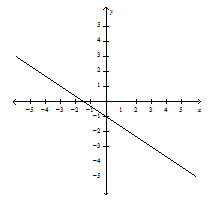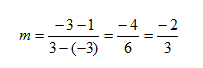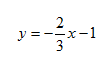Slope-Intercept Form

A rule for a function can be written by analyzing a graph. Using slope-intercept form, standard form, and point-slope form, functions can be written from a given graph.

To write a function in slope-intercept form given a graph, determine the slope and y-intercept of the graph and then substitute these values into the formula y = mx + b. If it is not possible to determine the y-intercept from the graph, determine a different point, substitute it into the equation and solve for b.

Example Find the function rule for the graph using the slope-intercept form.Step 1. Choose any two points to find slope. (-3, 1) & (3, -3)Step 2. Find the value of the y-intercept.

(0, -1)

b = -1

Step 3. Substitute the m and b values into y = mx + b to create the function rule.